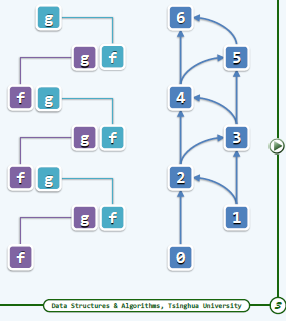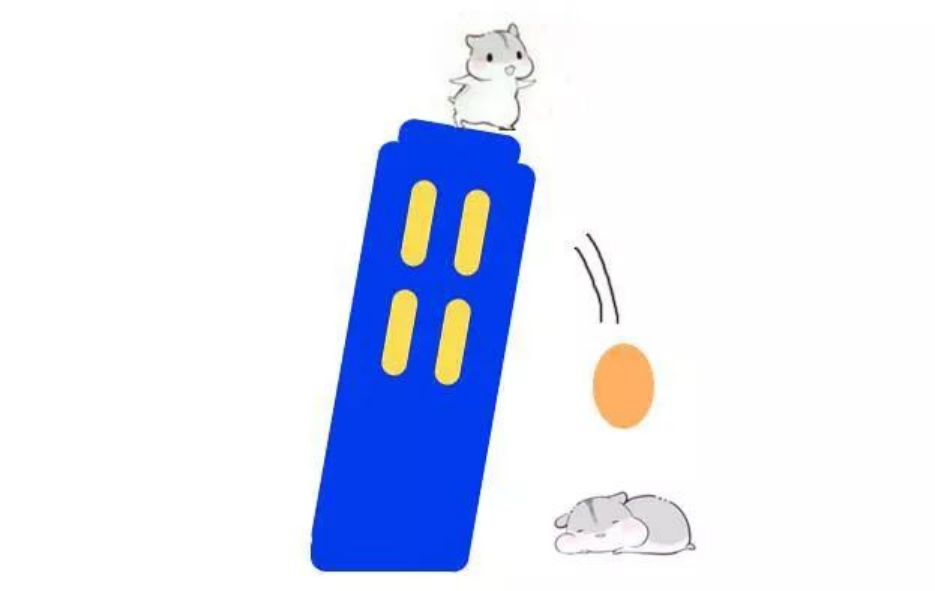# 【HDU 2013 猴子吃桃子】 尾递归与迭代

+关注继续查看

f(i) = sum(i)/2 + 1　　　　　　(1)

sum(i+1) = sum(i) - f(i)　　　 (2)

求sum(1)。

1 int sum(int x){
2     if(x==n) return 1;
3     return 2*sum(x+1) + 2;
4 }

1 ans=1;
2 int i=1;
3 while(i++ < n){
4     ans = 2*ans + 2;
5 }

1 int fib(int n){
2     if(n==0) return 0;
3     if(n==1) return 1;
4     return fib(n-1)+fib(n-2);
5 }

1 int fibI(int n){
2     int f=0, g=1;     // fib(0), fib(1)
3     int i=1;
4     while(i++ < n){
5         g = g + f;     // fib(n) = fib(n-1) + fib(n-2)
6         f = g - f;     // fib(n-1) = fib(n) - fib(n-2)
7     }
8     return g; // fib(n)
9 }47 0【兔年之兔子走迷宫】 用一个小游戏对回溯法进行实现 | C++

381 048 043 07-5 猴子吃桃问题
7-5 猴子吃桃问题 (20分) >一只猴子第一天摘下若干个桃子，当即吃了一半，还不过瘾，又多吃了一个；第二天早上又将剩下的桃子吃掉一半，又多吃了一个。以后每天早上都吃了前一天剩下的一半加一个。
93 0【刷穿 LeetCode】第 N 个泰波那契数 :「迭代」&「递归」&「矩阵快速幂」&「打表」
【刷穿 LeetCode】第 N 个泰波那契数 :「迭代」&「递归」&「矩阵快速幂」&「打表」
64 059 085 0160 0180 0
helena_wang
&quot;The master has failed more times than the beginner has even tried.&quot;